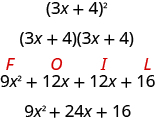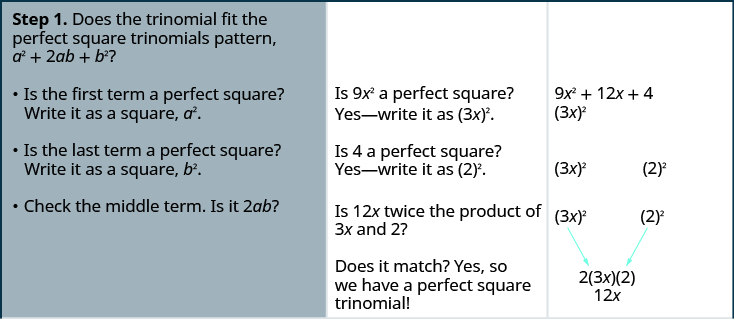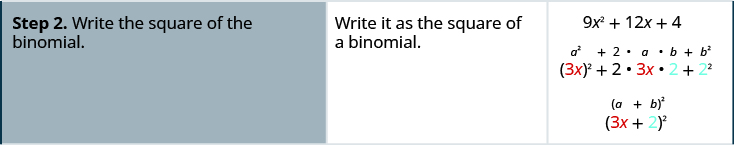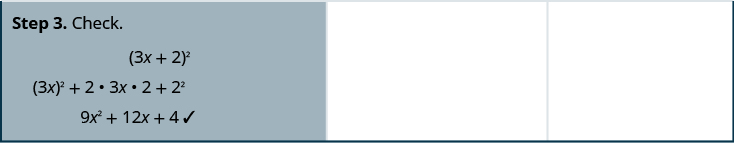# 7.4 Factor special products

 Page 1 / 7
By the end of this section, you will be able to:
• Factor perfect square trinomials
• Factor differences of squares
• Factor sums and differences of cubes
• Choose method to factor a polynomial completely

Before you get started, take this readiness quiz.

1. Simplify: ${\left(12x\right)}^{2}.$
If you missed this problem, review [link] .
2. Multiply: ${\left(m+4\right)}^{2}.$
If you missed this problem, review [link] .
3. Multiply: ${\left(p-9\right)}^{2}.$
If you missed this problem, review [link] .
4. Multiply: $\left(k+3\right)\left(k-3\right).$
If you missed this problem, review [link] .

The strategy for factoring we developed in the last section will guide you as you factor most binomials, trinomials, and polynomials with more than three terms. We have seen that some binomials and trinomials result from special products—squaring binomials and multiplying conjugates. If you learn to recognize these kinds of polynomials, you can use the special products patterns to factor them much more quickly.

## Factor perfect square trinomials

Some trinomials are perfect squares. They result from multiplying a binomial times itself. You can square a binomial by using FOIL, but using the Binomial Squares pattern you saw in a previous chapter saves you a step. Let’s review the Binomial Squares pattern by squaring a binomial using FOIL.The first term is the square of the first term of the binomial and the last term is the square of the last. The middle term is twice the product of the two terms of the binomial.

$\begin{array}{c}\hfill {\left(3x\right)}^{2}+2\left(3x·4\right)+{4}^{2}\hfill \\ \hfill 9{x}^{2}+24x+16\hfill \end{array}$

The trinomial 9 x 2 + 24 +16 is called a perfect square trinomial. It is the square of the binomial 3 x +4.

We’ll repeat the Binomial Squares Pattern here to use as a reference in factoring.

## Binomial squares pattern

If a and b are real numbers,

$\begin{array}{cccc}\hfill {\left(a+b\right)}^{2}={a}^{2}+2ab+{b}^{2}\hfill & & & \hfill \phantom{\rule{2em}{0ex}}{\left(a-b\right)}^{2}={a}^{2}-2ab+{b}^{2}\hfill \end{array}$

When you square a binomial, the product is a perfect square trinomial. In this chapter, you are learning to factor—now, you will start with a perfect square trinomial and factor it into its prime factors.

You could factor this trinomial using the methods described in the last section, since it is of the form ax 2 + bx + c . But if you recognize that the first and last terms are squares and the trinomial fits the perfect square trinomials pattern    , you will save yourself a lot of work.

Here is the pattern—the reverse of the binomial squares pattern.

## Perfect square trinomials pattern

If a and b are real numbers,

$\begin{array}{cccc}\hfill {a}^{2}+2ab+{b}^{2}={\left(a+b\right)}^{2}\hfill & & & \hfill \phantom{\rule{2em}{0ex}}{a}^{2}-2ab+{b}^{2}={\left(a-b\right)}^{2}\hfill \end{array}$

To make use of this pattern, you have to recognize that a given trinomial fits it. Check first to see if the leading coefficient is a perfect square, ${a}^{2}$ . Next check that the last term is a perfect square, ${b}^{2}$ . Then check the middle term—is it twice the product, 2 ab ? If everything checks, you can easily write the factors.

## How to factor perfect square trinomials

Factor: $9{x}^{2}+12x+4$ .

## SolutionFactor: $4{x}^{2}+12x+9$ .

${\left(2x+3\right)}^{2}$

Factor: $9{y}^{2}+24y+16$ .

${\left(3y+4\right)}^{2}$

The sign of the middle term determines which pattern we will use. When the middle term is negative, we use the pattern ${a}^{2}-2ab+{b}^{2}$ , which factors to ${\left(a-b\right)}^{2}$ .

The steps are summarized here.

## Factor perfect square trinomials.

$\begin{array}{ccccccc}\mathbf{\text{Step 1.}}\phantom{\rule{0.2em}{0ex}}\text{Does the trinomial fit the pattern?}\hfill & & & \hfill {a}^{2}+2ab+{b}^{2}\hfill & & & \hfill \phantom{\rule{2em}{0ex}}{a}^{2}-2ab+{b}^{2}\hfill \\ \phantom{\rule{2.5em}{0ex}}•\phantom{\rule{0.5em}{0ex}}\text{Is the first term a perfect square?}\hfill & & & \hfill {\left(a\right)}^{2}\hfill & & & \hfill \phantom{\rule{2em}{0ex}}{\left(a\right)}^{2}\hfill \\ \phantom{\rule{4em}{0ex}}\text{Write it as a square.}\hfill & & & & & & \\ \phantom{\rule{2.5em}{0ex}}•\phantom{\rule{0.5em}{0ex}}\text{Is the last term a perfect square?}\hfill & & & {\left(a\right)}^{2}\phantom{\rule{4.5em}{0ex}}{\left(b\right)}^{2}\hfill & & & \phantom{\rule{2em}{0ex}}{\left(a\right)}^{2}\phantom{\rule{4.5em}{0ex}}{\left(b\right)}^{2}\hfill \\ \phantom{\rule{4em}{0ex}}\text{Write it as a square.}\hfill & & & & & & \\ \phantom{\rule{2.5em}{0ex}}•\phantom{\rule{0.5em}{0ex}}\text{Check the middle term. Is it}\phantom{\rule{0.2em}{0ex}}2ab?\hfill & & & {\left(a\right)}^{2}{}_{\text{↘}}\underset{2·a·b}{}{}_{\text{↙}}{\left(b\right)}^{2}\hfill & & & \phantom{\rule{2em}{0ex}}{\left(a\right)}^{2}{}_{\text{↘}}\underset{2·a·b}{}{}_{\text{↙}}{\left(b\right)}^{2}\hfill \\ \mathbf{\text{Step 2.}}\phantom{\rule{0.2em}{0ex}}\text{Write the square of the binomial.}\hfill & & & \hfill {\left(a+b\right)}^{2}\hfill & & & \hfill \phantom{\rule{2em}{0ex}}{\left(a-b\right)}^{2}\hfill \\ \mathbf{\text{Step 3.}}\phantom{\rule{0.2em}{0ex}}\text{Check by multiplying.}\hfill & & & & & & \end{array}$

#### Questions & Answers

find the solution to the following functions, check your solutions by substitution. f(x)=x^2-17x+72
Carlos Reply
Aziza is solving this equation-2(1+x)=4x+10
Sechabe Reply
No. 3^32 -1 has exactly two divisors greater than 75 and less than 85 what is their product?
KAJAL Reply
x^2+7x-19=0 has Two solutions A and B give your answer to 3 decimal places
Adedamola Reply
please the answer to the example exercise
Patricia Reply
3. When Jenna spent 10 minutes on the elliptical trainer and then did circuit training for20 minutes, her fitness app says she burned 278 calories. When she spent 20 minutes onthe elliptical trainer and 30 minutes circuit training she burned 473 calories. How manycalories does she burn for each minute on the elliptical trainer? How many calories doesshe burn for each minute of circuit training?
Edwin Reply
.473
Angelita
?
Angelita
John left his house in Irvine at 8:35 am to drive to a meeting in Los Angeles, 45 miles away. He arrived at the meeting at 9:50. At 3:30 pm, he left the meeting and drove home. He arrived home at 5:18.
DaYoungan Reply
p-2/3=5/6 how do I solve it with explanation pls
Adedamola Reply
P=3/2
Vanarith
1/2p2-2/3p=5p/6
James
don't understand answer
Cindy
4.5
Ruth
is y=7/5 a solution of 5y+3=10y-4
Adedamola Reply
yes
James
don't understand answer
Cindy
Lucinda has a pocketful of dimes and quarters with a value of $6.20. The number of dimes is 18 more than 3 times the number of quarters. How many dimes and how many quarters does Lucinda have? Rhonda Reply Find an equation for the line that passes through the point P ( 0 , − 4 ) and has a slope 8/9 . Gabriel Reply is that a negative 4 or positive 4? Felix y = mx + b Felix if negative -4, then -4=8/9(0) + b Felix -4=b Felix if positive 4, then 4=b Felix then plug in y=8/9x - 4 or y=8/9x+4 Felix Macario is making 12 pounds of nut mixture with macadamia nuts and almonds. macadamia nuts cost$9 per pound and almonds cost $5.25 per pound. how many pounds of macadamia nuts and how many pounds of almonds should macario use for the mixture to cost$6.50 per pound to make?
Cherry Reply
Nga and Lauren bought a chest at a flea market for $50. They re-finished it and then added a 350 % mark - up Makaila Reply$1750
Cindy
the sum of two Numbers is 19 and their difference is 15
Abdulai Reply
2, 17
Jose
interesting
saw
4,2
Cindy
Felecia left her home to visit her daughter, driving 45mph. Her husband waited for the dog sitter to arrive and left home 20 minutes, or 13 hour later. He drove 55mph to catch up to Felecia. How long before he reaches her?
Rafi Reply
hola saben como aser un valor de la expresión
NAILEA

### Read also:

#### Get Jobilize Job Search Mobile App in your pocket Now!

Source:  OpenStax, Elementary algebra. OpenStax CNX. Jan 18, 2017 Download for free at http://cnx.org/content/col12116/1.2
Google Play and the Google Play logo are trademarks of Google Inc.

Notification Switch

Would you like to follow the 'Elementary algebra' conversation and receive update notifications?By John GabrieliBy OpenStaxBy Madison ChristianBy OpenStaxBy Mike WolfBy Sandhills MLTBy OpenStaxBy Rebecca ButterfieldBy OpenStaxBy Rhodes